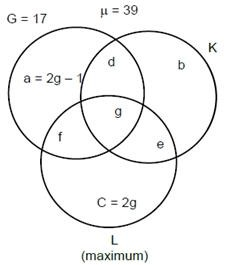### CAT 2018 – Slot 2 – Logical Reasoning and Data Interpretation – Fun Sports (FS) provides training in three sports – Gilli-danda (G), Kho-Kho (K), and Ludo (L)

##### Fun Sports (FS) provides training in three sports – Gilli-danda (G), Kho-Kho (K), and Ludo (L). Currently it has an enrollment of 39 students each of whom is enrolled in at least one of the three sports. The following details are known: 1. The number of students enrolled only in L is double the number of students enrolled in all the three sports. 2. There are a total of 17 students enrolled in G. 3. The number of students enrolled only in G is one less than the number of students enrolled only in L. 4. The number of students enrolled only in K is equal to the number of students who are enrolled in both K and L. 5. The maximum student enrollment is in L. 6. Ten students enrolled in G are also enrolled in at least one more sport.

Q. 1: What is the minimum number of students enrolled in both G and L but not in K?

##### Q. 2: If the numbers of students enrolled in K and L are in the ratio 19:22, then what is the number of students enrolled in L?1. 182. 193. 174. 22

Q. 3: Due to academic pressure, students who were enrolled in all three sports were asked to withdraw from one of the three sports. After the withdrawal, the number of students enrolled in G was six less than the number of students enrolled in L, while the number of students enrolled in K went down by one. After the withdrawal, how many students were enrolled in both G and K?

Q. 4: Due to academic pressure, students who were enrolled in all three sports were asked to withdraw from one of the three sports. After the withdrawal, the number of students enrolled in G was six less than the number of students enrolled in L, while the number of students enrolled in K went down by one. After the withdrawal, how many students were enrolled in both G and L?
1. 6
2. 7
3. 5
4. 8

The given data can be represented as follows.f + g + d =10 (given) g + e = b (given)
Since f + g + d = 10, g = 7 = 2g – 1 Therefore, 2g = 8  f = 4
Thus, g = 4, c = 8, a = 7 and f + d = 6
b + e = 39 – (G + c) = 14
therefore g + 2e = 14 Hence, e = 5 and b = 9 Since, L is maximum we get the following cases. Case (i)
G = 17 K = 20 L = 21 d = 2 f = 4

Case (ii)
G = 17 K = 19 L = 22 d = 1 f = 5
Case (iii)
G = 17 K = 18 L = 23 d = 0 f = 6

Question 1:

G and L but not K = f = 4. Ans : 4

Question 2:

The given condition is possible in case (ii). Hence, the number of students enrolled in L = 22.
Ans : 22

question 3:

From g = 4, one person moves to f, one person to d and two persons to e. Then the value of G and K = d + g = 2. Ans : 2

Question 4:
From the above G and L = f = 6. Ans : 6

##### Checkout Other Questions of CAT 2018 Slot 2 Paper:

Verbal Ability :              |   Q.01- Q.05  |  Q.06- Q.9  |  Q.10- Q.14  |  Q.15- Q.19  |  Q.20- Q.24  |  Q.25- Q.29  |  Q.30 – Q.34  |

Logical Reasoning :    |   Q.29 – Q.32  |

Quantitative Aptitude: |